Question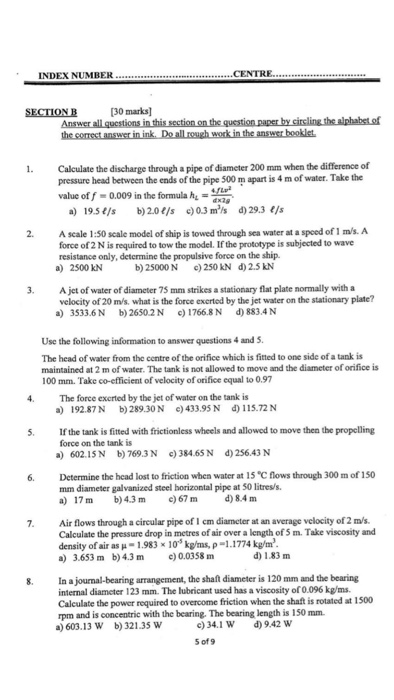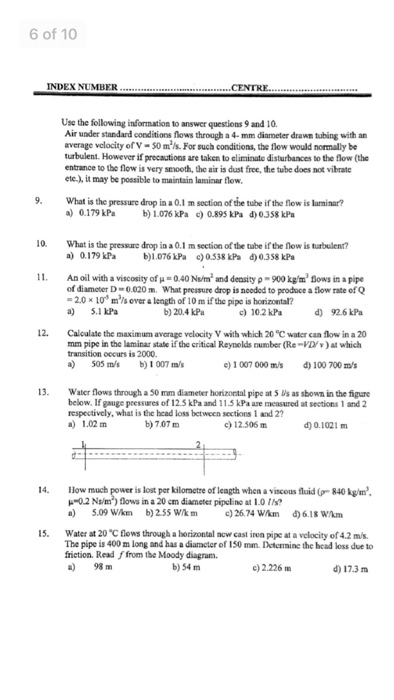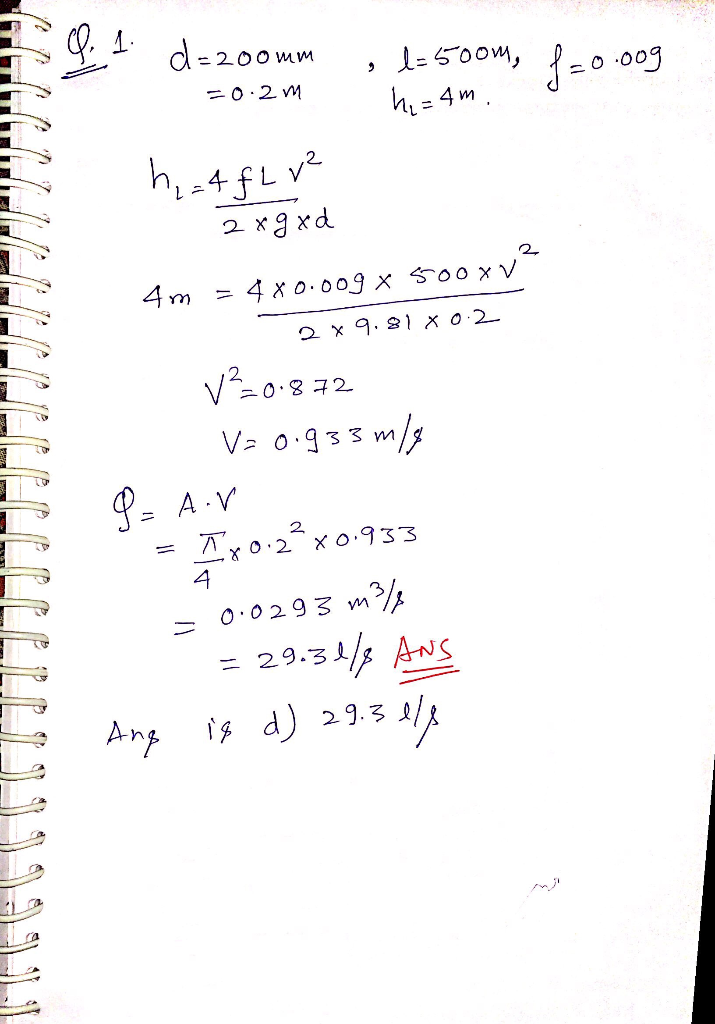#### Earn Coins

Coins can be redeemed for fabulous gifts.

Similar Homework Help Questions
• ### Question 3 (a) Water flows through a horizontal pipeline of constant 400 mm diameter in a...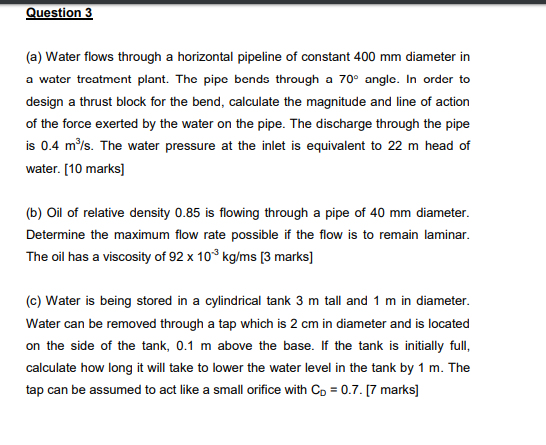Question 3 (a) Water flows through a horizontal pipeline of constant 400 mm diameter in a water treatment plant. The pipe bends through a 70° angle. In order to design a thrust block for the bend, calculate the magnitude and line of action of the force exerted by the water on the pipe. The discharge through the pipe is 0.4 m/s. The water pressure at the inlet is equivalent to 22 m head of water. [10 marks] (b) Oil of...

• ### Question 6 A water jet discharges from a water tank through a 100 mm diameter pipe...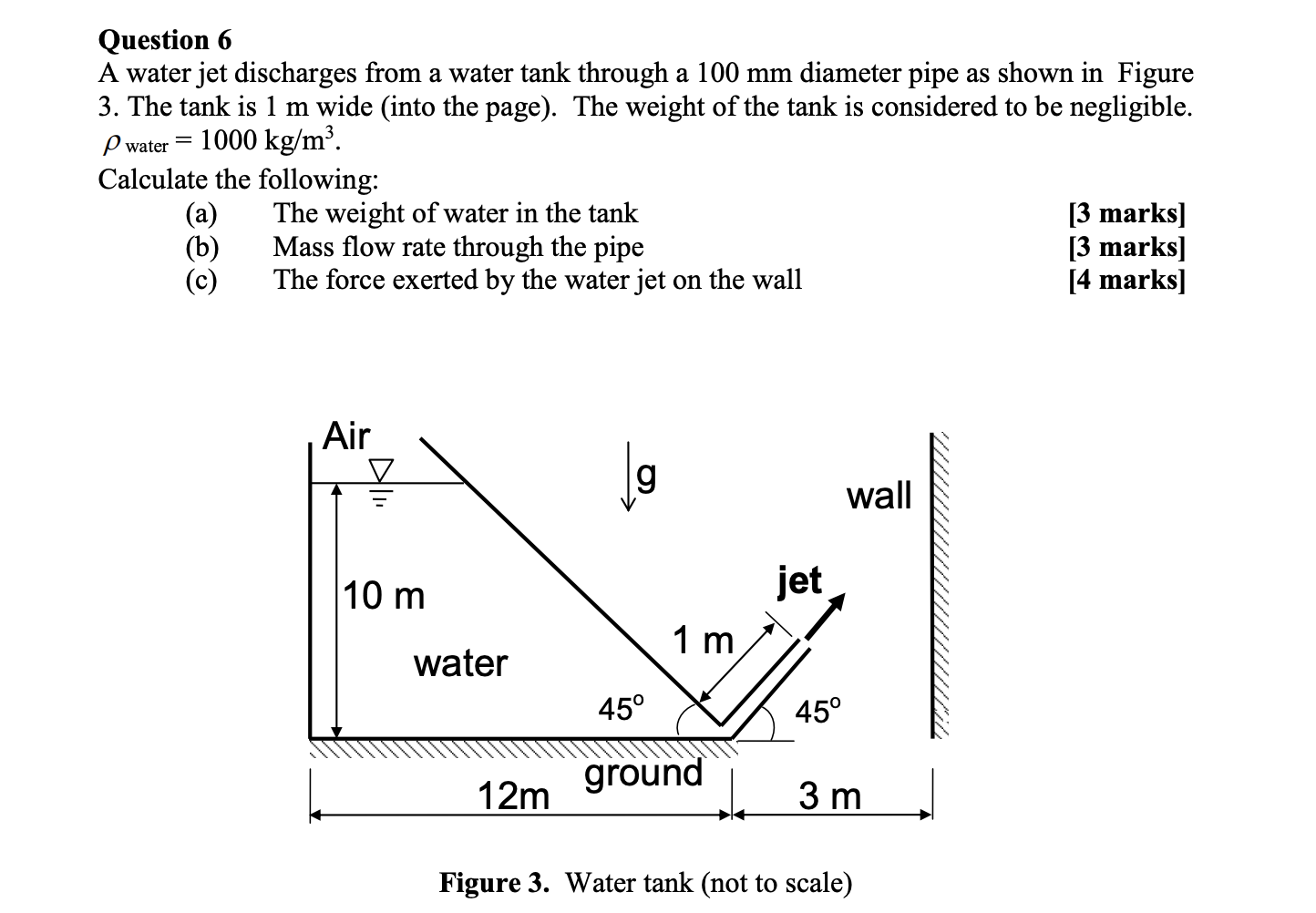Question 6 A water jet discharges from a water tank through a 100 mm diameter pipe as shown in Figure 3. The tank is 1 m wide (into the page). The weight of the tank is considered to be negligible. Pwater 1000 kg/m3. Calculate the following: The weight of water in the tank [3 marks] (b) Mass flow rate through the pipe [3 marks) (c) The force exerted by the water jet on the wall [4 marks] Air wall 10...

• ### Problem 1. Water flows from a large tank through a smooth pipe of length 80 m....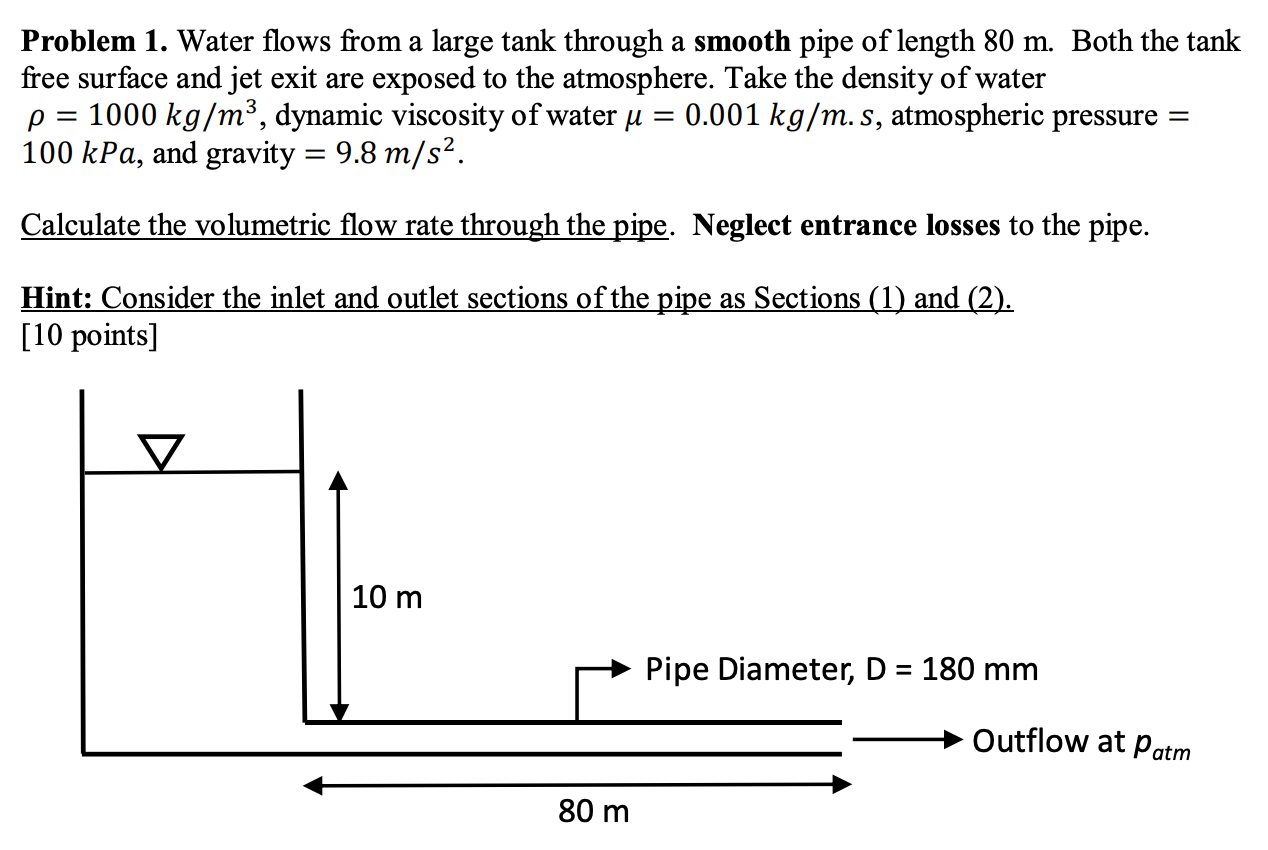Problem 1. Water flows from a large tank through a smooth pipe of length 80 m. Both the tank free surface and jet exit are exposed to the atmosphere. Take the density of water p = 1000 kg/m3, dynamic viscosity of water u = 0.001 kg/m.s, atmospheric pressure = 100 kPa, and gravity = 9.8 m/s2. Calculate the volumetric flow rate through the pipe. Neglect entrance losses to the pipe. Hint: Consider the inlet and outlet sections of the pipe...

• ### Problem 1. Water flows from a large tank through a smooth pipe of length 80 m....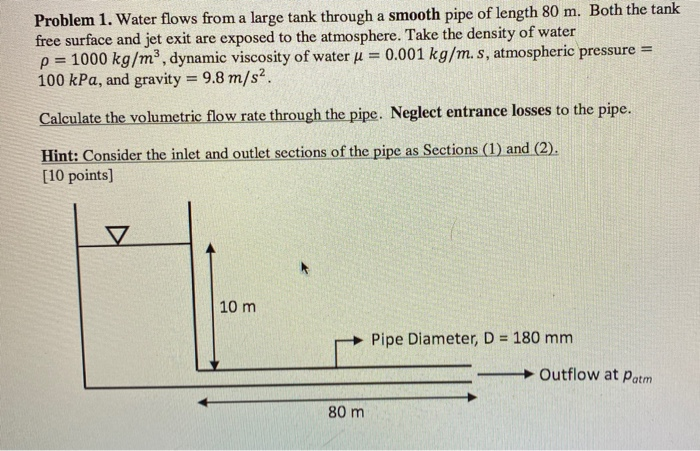Problem 1. Water flows from a large tank through a smooth pipe of length 80 m. Both the tank free surface and jet exit are exposed to the atmosphere. Take the density of water p = 1000 kg/m3, dynamic viscosity of water j = 0.001 kg/m.s, atmospheric pressure = 100 kPa, and gravity = 9.8 m/s2. Calculate the volumetric flow rate through the pipe. Neglect entrance losses to the pipe. Hint: Consider the inlet and outlet sections of the pipe...

• ### Question in the picture smooth pipe: Total length, L Diameter, d -25 mm 4 m tank...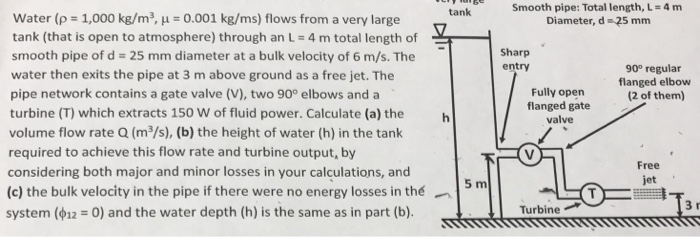Question in the picture smooth pipe: Total length, L Diameter, d -25 mm 4 m tank water (pa 1,000 kg/m3, μ 0.001 kg/ms) flows from a very large tank (that is open to atmosphere) through an L 4 m total length of smooth pipe of d = 25 mm diameter at a bulk velocity of 6 m/s. The water then exits the pipe at 3 m above ground as a free jet. The pipe network contains a gate valve (V),...

• ### A flow nozzle equipped with a differential pressure gage is used to measure the flow rate of water at 10°C (p 9997 kg/m3 and p 1.307 x 10-3 kg/m s) through a 3-cm-diameter horizontal pipe. The nozzle...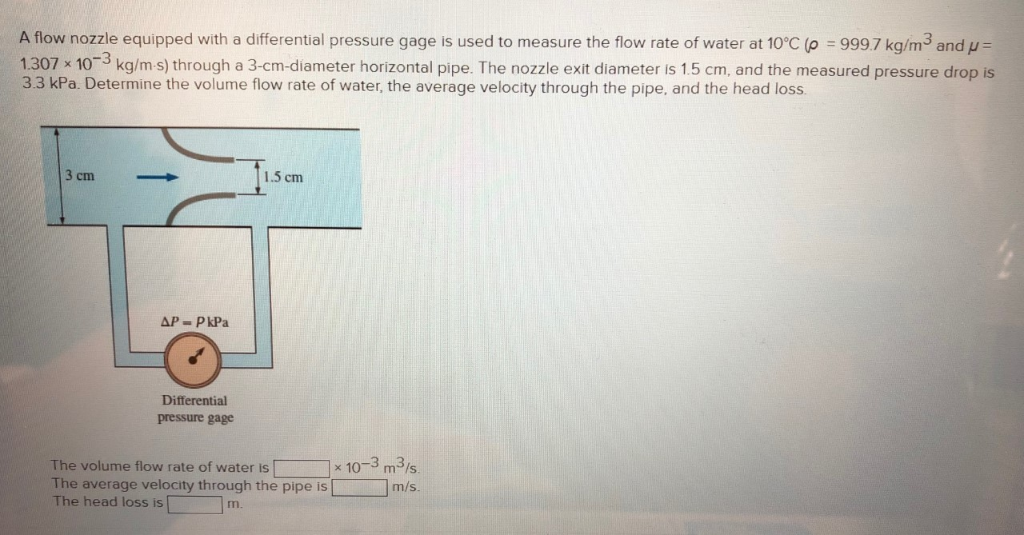A flow nozzle equipped with a differential pressure gage is used to measure the flow rate of water at 10°C (p 9997 kg/m3 and p 1.307 x 10-3 kg/m s) through a 3-cm-diameter horizontal pipe. The nozzle exit diameter is 1.5 cm, and the measured pressure drop is 3.3 kPa. Determine the volume flow rate of water, the average velocity through the pipe, and the head loss 1.5 cm Differential pressure gage -3 m3(s m/s The volume flow rate of...

• ### QUESTION 1 Water at 10 °C is flowing in a 90 bend pipe, with inner diameter...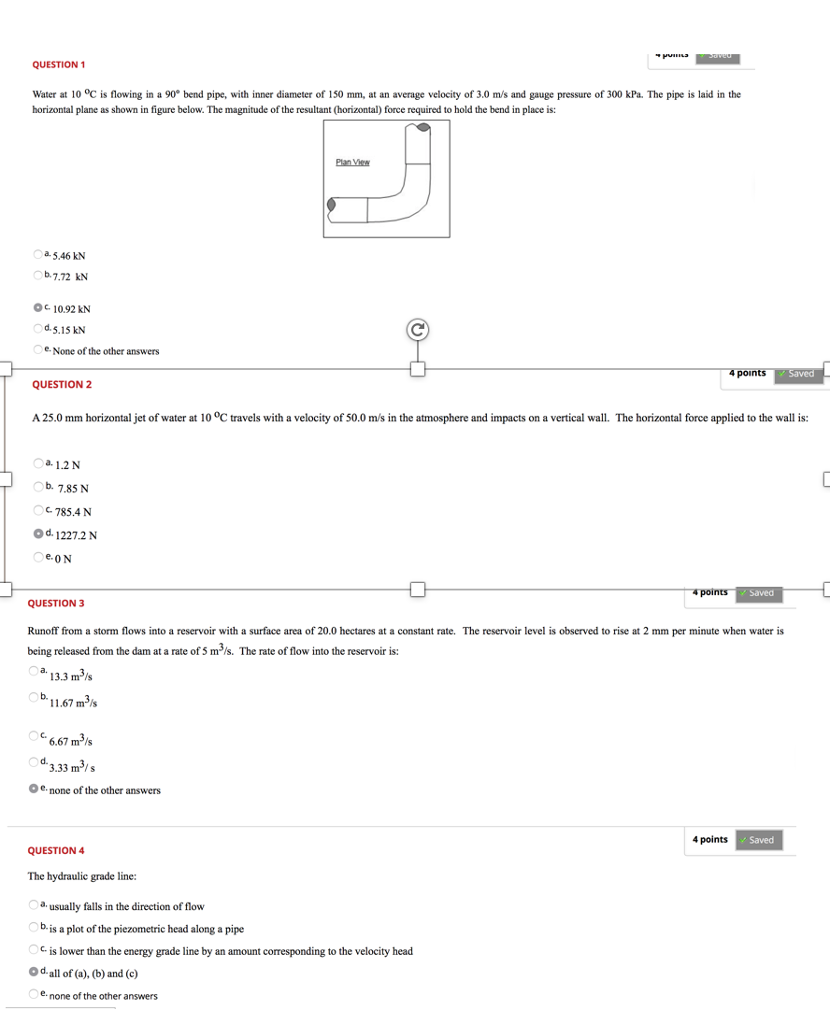QUESTION 1 Water at 10 °C is flowing in a 90 bend pipe, with inner diameter of 150 mm, at an average velocity of 3.0 m/s and gauge pressure of 300 kPa. The pipe is laid in the horizontal plane as shown in figure below. The magnitude of the resultant (horizontal) force required to hold the bend in place is: a 5.46 kN b.7.72 kN Oc 10.92 kN d.5.15 kN . None of the other answers QUESTION 2 A 25.0...

• ### 6-7. Oil flows through the 100-mm-diameter pipe with a velocity of 8 m/s. If the pressure...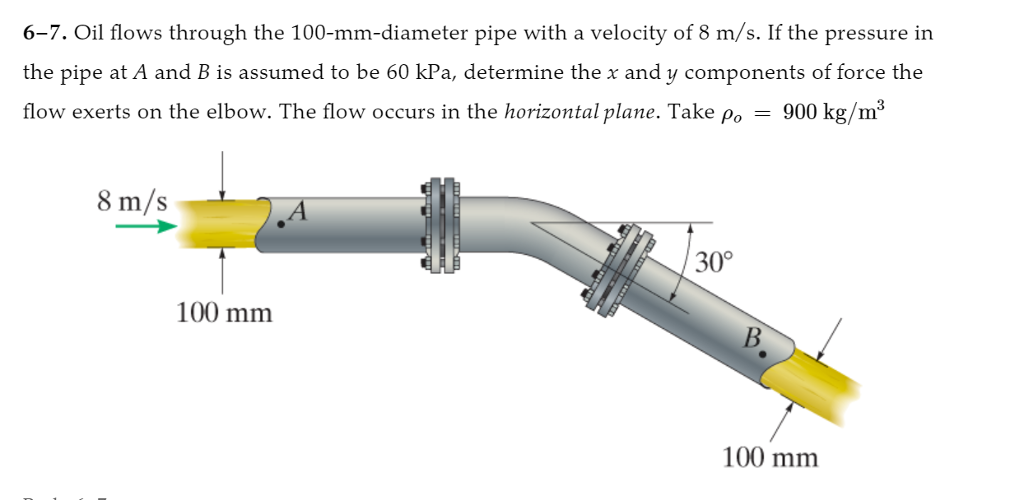6-7. Oil flows through the 100-mm-diameter pipe with a velocity of 8 m/s. If the pressure in the pipe at A and B is assumed to be 60 kPa, determine the x and y components of force the flow exerts on the elbow. The flow occurs in the horizontal plane. Take ρο -900 kg/m3 8 m/s 30° 100 mm 100 mm

• ### Q2: (20pts) Water flow in a pipe with an orifice inside where the diameter of the...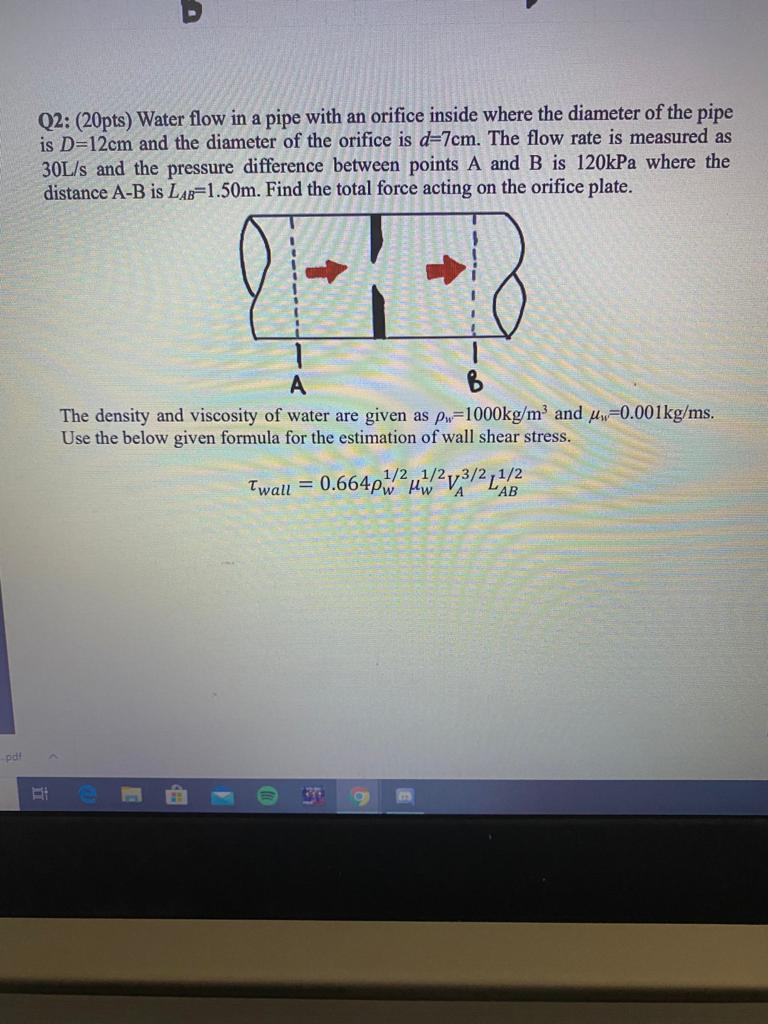Q2: (20pts) Water flow in a pipe with an orifice inside where the diameter of the pipe is D=12cm and the diameter of the orifice is d=7cm. The flow rate is measured as 30L/s and the pressure difference between points A and B is 120kPa where the distance A-B is LAB=1.50m. Find the total force acting on the orifice plate. The density and viscosity of water are given as pw=1000kg/m and Uw=0.001kg/ms. Use the below given formula for the estimation...

• ### 1) Water is pumped steadily through a 0.10 m diameter pipe from one closed, pressurized tank...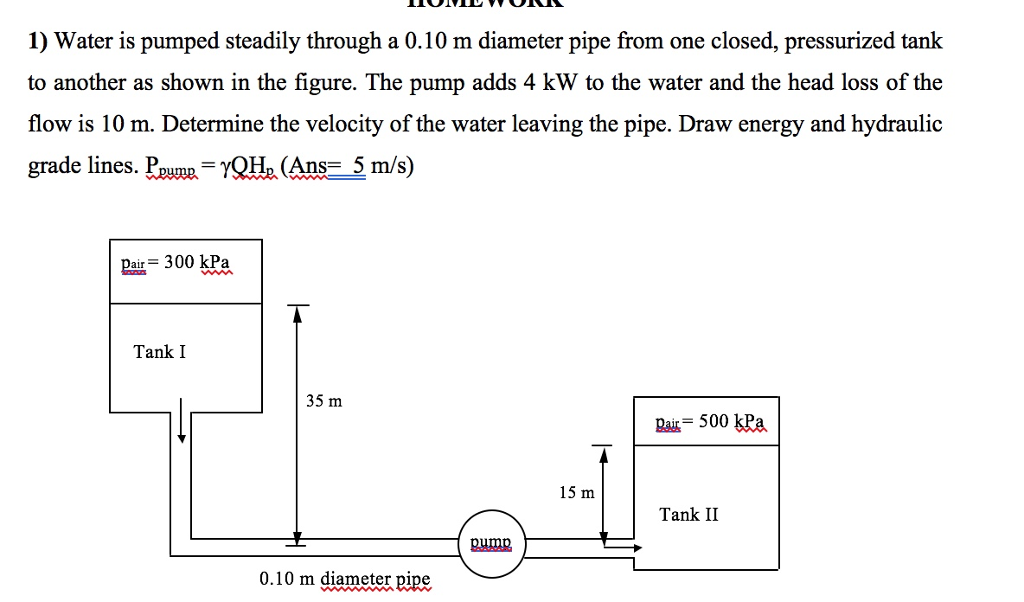1) Water is pumped steadily through a 0.10 m diameter pipe from one closed, pressurized tank to another as shown in the figure. The pump adds 4 kW to the water and the head loss of the flow is 10 m. Determine the velocity of the water leaving the pipe. Draw energy and hydraulic grade lines. Ppupn= A(Ans= 5m/s) ar 300 kPa Tank I 35 m pa = 500 kPa 15 m Tank II DUMD 0.10 m diameter pipe